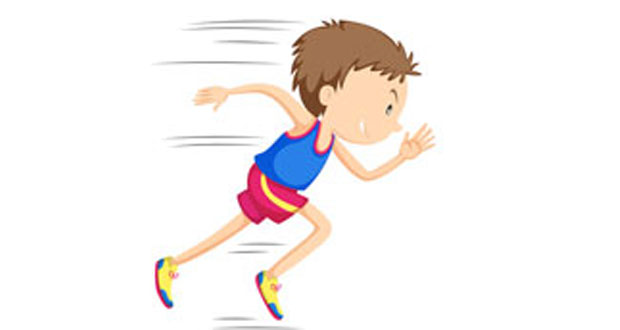# Speed and Velocity in Sports BiomechanicsHere we explain the difference between distance and displacement, vector and scalar quantities as well as speed and velocity in sport and how to do calculations.

## Distance and displacement

What is the difference between distance and displacement? Displacement is how far away a body has moved from its starting point. It is the shortest distance between the starting point and the finishing point.

For example, a sailing Yacht might zig-zag (tack) backward and forward in order to sail 100m East. Even though the total distance travelled by the yacht may be much greater than 100m, the displacement of the Yacht is still only 100m. The units of both distance and displacement are metres (m).

More examples of distance and displacement:

• A marathon runner has run a distance of approximately 26 miles 352 metres. Is displacement the same? Probably not unless the course is one very straight line.
• A 400m sprinter? The distance travelled by the sprinter is 400m, but the displacement is practically zero as they finish in the same place they started.

## Speed and velocity

Speed and velocity are used to describe how fast something is going. Speed is the rate of change of distance and is a scalar quantity.

Speed (ms-1) = Distance (m) / Time (s)

Velocity is the speed of a body in a specific direction and is the rate of change of displacement. Unlike speed, velocity is a vector quantity which means it has a direction as well as a magnitude. So if the direction of the moving body changes then the velocity changes, even though the speed might stay the same.

Velocity (m/s) = Displacement (m) / Time (s)